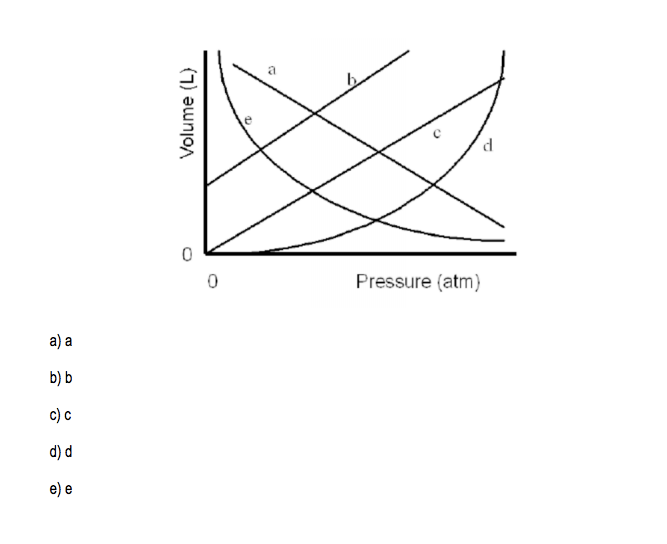# Problem: Which of the lines on the figure below is the best representation of the relationship between the volume of a gas and its pressure, other factors remaining constant?

###### FREE Expert Solution
84% (274 ratings)
###### Problem Details

Which of the lines on the figure below is the best representation of the relationship between the volume of a gas and its pressure, other factors remaining constant?What scientific concept do you need to know in order to solve this problem?

Our tutors have indicated that to solve this problem you will need to apply the The Ideal Gas Law Applications concept. You can view video lessons to learn The Ideal Gas Law Applications. Or if you need more The Ideal Gas Law Applications practice, you can also practice The Ideal Gas Law Applications practice problems.

What is the difficulty of this problem?

Our tutors rated the difficulty ofWhich of the lines on the figure below is the best represent...as medium difficulty.

How long does this problem take to solve?

Our expert Chemistry tutor, Jules took 2 minutes and 32 seconds to solve this problem. You can follow their steps in the video explanation above.

What professor is this problem relevant for?

Based on our data, we think this problem is relevant for Professor Sumner's class at UF.